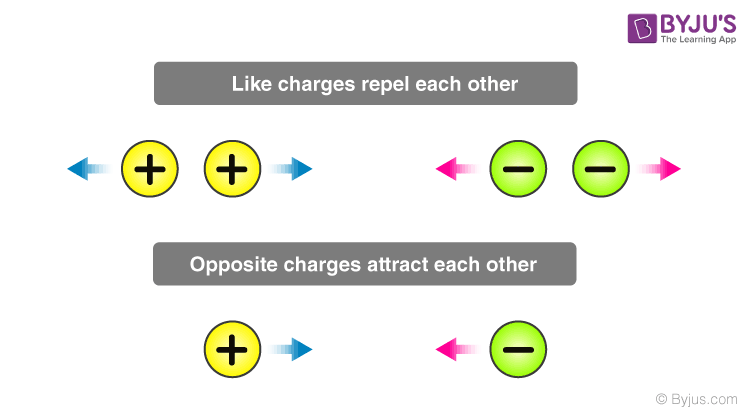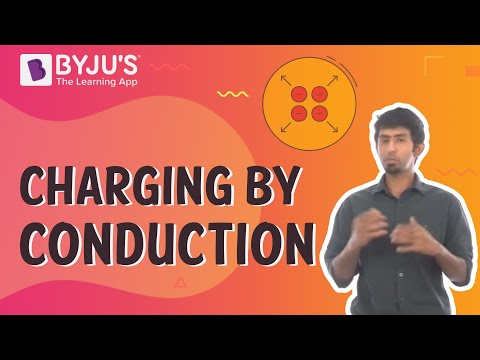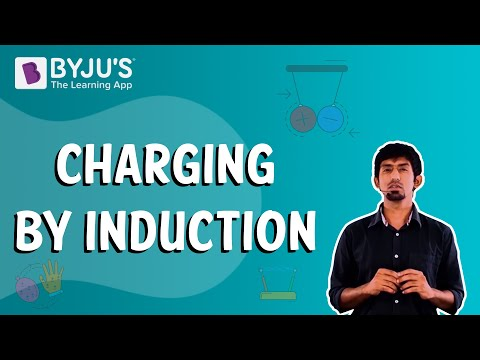# Electric Charge

Electric charge is a fundamental concept within physics, serving as a cornerstone for comprehending the physical world. This article will delve into the intricate nature of the electric charge and its accompanying properties. We will unveil the essence of electric charge through meticulous examination, providing a comprehensive definition and exploring its different types and governing principles. Additionally, our article will encompass a comprehensive overview of the various methodologies employed in the charging process, including friction, conduction, and induction.

## What is an Electric Charge?

In the CBSE curriculum, understanding the concept of electric charge and its various types is of utmost importance.

### Electric Charge Definition

Electric charge can be defined as a fundamental property of subatomic particles that gives rise to the phenomenon of experiencing force in the presence of electric and magnetic fields. These fields exert influence on charged particles, resulting in observable effects.

### Types of Electric Charge

Electric charge comes in two main types: positive and negative charges. Positive charges are associated with protons, which are subatomic particles residing in the nucleus of an atom. They are represented by the symbol “+”. On the other hand, negative charges are linked to electrons, which orbit the atomic nucleus and are denoted by the symbol “-“.

The distinction between positive and negative charges plays a vital role in comprehending the behaviour of electrically charged objects. Opposite charges, such as positive and negative, attract each other, while like charges, such as positive and positive or negative and negative, repel each other. This fundamental principle is the foundation for various concepts in electromagnetism and is pivotal in understanding the interaction of charged particles.When an object carries a negative charge, it possesses an excess of electrons compared to protons. Conversely, a positive charge indicates an excess of protons relative to electrons.

It’s important to note that when an equal number of positive and negative charges are present, they cancel each other out, resulting in a neutral state for the object.

By grasping the definition of electric charge and recognizing the significance of positive and negative charges, one can understand the fundamental principles governing electricity and magnetism.

Note: In the context of electric charge, the terms “attraction” and “repulsion” are used to describe how charges interact with each other.

### Is Electric Charge a Vector Quantity?

No, electric charge is not a vector quantity; it is a scalar quantity. While vectors have both magnitude and direction and obey vector addition laws like the triangle law and parallelogram law, electric charge does not exhibit these properties. When currents meet at a junction, the resulting current is determined by the algebraic sum of the individual currents rather than their vector sum. Thus, electric charge is considered a scalar quantity, despite having magnitude and direction.

## Measuring Electric Charge

Coloumb is the unit of electric charge.

“One coulomb is the quantity of charge transferred in one second.”

Mathematically, the definition of a coloumb is represented as:

Q = I.t

In the equation, Q is the electric charge, I is the electric current and t is the time.

## Properties of Electric Charge

Electric charge possesses several important properties that help us understand its behaviour. Let’s explore these properties:

When charges combine, their magnitudes add up algebraically. For example, if we have a positive charge of +3 units and a negative charge of -2 units, the resulting charge would be +1 unit.

### Conservation of Electric Charge:

In an isolated system, electric charge is conserved. This means that the total electric charge within the system remains constant over time. The algebraic sum of all the charges present in the system remains the same.

### Quantization of Electric Charge

Electric charge comes in discrete, indivisible units called elementary charges. The smallest unit of electric charge is the charge carried by an electron, which is approximately -1.6 x 10-19 coulombs. This quantization of charge implies that electric charge cannot be divided into smaller parts.

Understanding these properties helps us comprehend the behaviour of electric charges and their importance in various scientific phenomena.

Note: “Algebraic sum” refers to adding charges, considering their signs (+ or -).

To understand the properties of charge in detail, read the article below:

## Coulomb’s Law

We know that like charges repel each other, while unlike charges attract. However, have you ever wondered about the strength of these forces acting between charges? Coulomb’s Law offers us a method to calculate this force precisely.

According to Coulomb’s Law, the magnitude of the electrostatic force between two point charges is directly proportional to the product of their magnitudes and inversely proportional to the square of the distance separating them.

The Coulomb’s Law is given by the expression:

$$\begin{array}{l}F_e = \frac{kq_1q_2}{r^2}\end{array}$$

where Fe is the electric force, q1 and q2 are electric charges, k is the Coulomb’s constant 8.988×109 N⋅m2/C2 and r is the distance of separation.

By applying Coulomb’s Law, we can quantitatively determine the strength of the electric force between charges and gain valuable insights into their interactions. This fundamental principle holds great significance in the field of electromagnetism and enables us to analyse various electrical phenomena.

Through the application of Coulomb’s Law, scientists and researchers have been able to uncover the intricate workings of electric forces and comprehend their profound impact on the world around us.

## Methods of Charging

The process of supplying electric charge to an object or causing it to lose electric charge is referred to as charging. There are three distinct methods by which an initially uncharged object can acquire charge:

• Charging by friction ( triboelectric charging)
• Charging by conduction
• Charging by induction

### Charging by Friction

When two objects are rubbed against each other, a transfer of charge occurs. In this process, one of the objects loses electrons while the other gains electrons. The object losing electrons becomes positively charged, while the object gaining electrons becomes negatively charged. This phenomenon, where both objects become charged due to friction, is commonly known as electrification by friction.

### Charging by Conduction

Charging by conduction involves bringing an uncharged object in close proximity to a charged object. If the charged object has an unequal number of protons and electrons, the uncharged object will discharge electrons to achieve stability. This transfer of charge through contact is known as charging by conduction.

### Charging by Induction

Charging by induction refers to the process of charging an uncharged object by merely bringing it close to a charged object, without any direct physical contact. Through induction, the charged object induces a redistribution of charges in the uncharged object, resulting in the acquisition of charge.

By understanding these different methods of charging, we can explore the fascinating ways in which objects become charged through friction, contact, or proximity. The study of charging provides valuable insights into the behaviour and interaction of electric charges in various scenarios.

#### Charging By Conduction#### Charging By Induction### Overview of Electric Charge

 Definition Electric Charge is the property of subatomic particles that causes it to experience a force when placed in an electromagnetic field. Symbol Q Formula Q = I.t SI Unit Coulomb Other Units Faraday, Ampere-Hour

## Frequently Asked Questions – FAQs

Q1

### What is electric charge?

Electric charge is a fundamental property of matter. It refers to the intrinsic property of particles that gives rise to electric forces and interactions.
Q2

### How are electric charges distributed within the atom?

Within an atom, electric charges are distributed among subatomic particles. Protons, found in the nucleus, carry positive charges, while electrons, which orbit the nucleus, carry negative charges. Neutrons, also present in the nucleus, have no electric charge.
Q3

### What are the positively charged subatomic particles called?

The positively charged subatomic particles are called protons. They have a positive electric charge.
When the matter has more protons than electrons, then it is said to have a positive charge.
Q4

### When will an electric charge be negative?

An electric charge will be negative when an object has an excess of electrons.
Q5

### Why is an electric charge a scalar quantity?

When two currents meet at a junction, the resultant current of these will be an algebraic sum and not the vector sum. Therefore, an electric current is a scalar quantity.
Q6

### What is the unit for measuring electric charge?

The unit for measuring electric charge is the coulomb (C). It is named after the French physicist Charles-Augustin de Coulomb.
Q7

### Define one coulomb.

One coulomb is the quantity of charge transferred in one second.
Q8

### What are the types of electric charges?

The two types of electric charges are positive and negative charges. Positive charges are associated with protons, while negative charges are associated with electrons.
Q9

### How is an uncharged object charged?

An uncharged object can be charged through various methods, such as charging by friction (rubbing two objects together), charging by conduction (contact with a charged object), or charging by induction (bringing an uncharged object close to a charged object without direct contact).
Q10

### What are the other units of electric charge?

Faraday and Ampere-Hour are the other units of electric charge. Apart from the coulomb, smaller units of electric charge include the microcoulomb (μC), nanocoulomb (nC), and picocoulomb (pC). These units are used to measure smaller quantities of electric charge.

## The following video serves as a helpful resource for reviewing the Electricity chapter in Class 10.Stay tuned to BYJU’S and Fall in Love with Learning!

Test your knowledge on Electric Charge